• 利用Nevanlinna理论研究了全平面内随机Dirichlet级数所表示的整函数的增长性和值分布，得到全平面内水平带形上的几个新的定理，推广了以往研究半平面内水平半带形上关于增长性和值分布的一些相关结论．
• 利用映射的不动点以及不动点阶的思想将整数环Z上的Fermat小定理推广到一般集合S上,并运用该推广讨论了Dirichlet定理的一种特殊情形:只要给定正整数m≥3,那么算术数列1+l m(l =0 ,1 ,2 ,…)中一定存在无穷多个素数.
• 机器学习中常遇到关于各种分布的问题，不过这些知识都已经忘得差不多了，就搜了点资料，详细讲解下伯努利分布、二项分布、多项分布、Beta分布、Dirichlet分布 ，用于后期回顾。
机器学习中常遇到关于各种分布的问题，不过这些知识都已经忘得差不多了，就搜了点资料，详细讲解下伯努利分布、二项分布、多项分布、Beta分布、Dirichlet分布 ，用于后期回顾。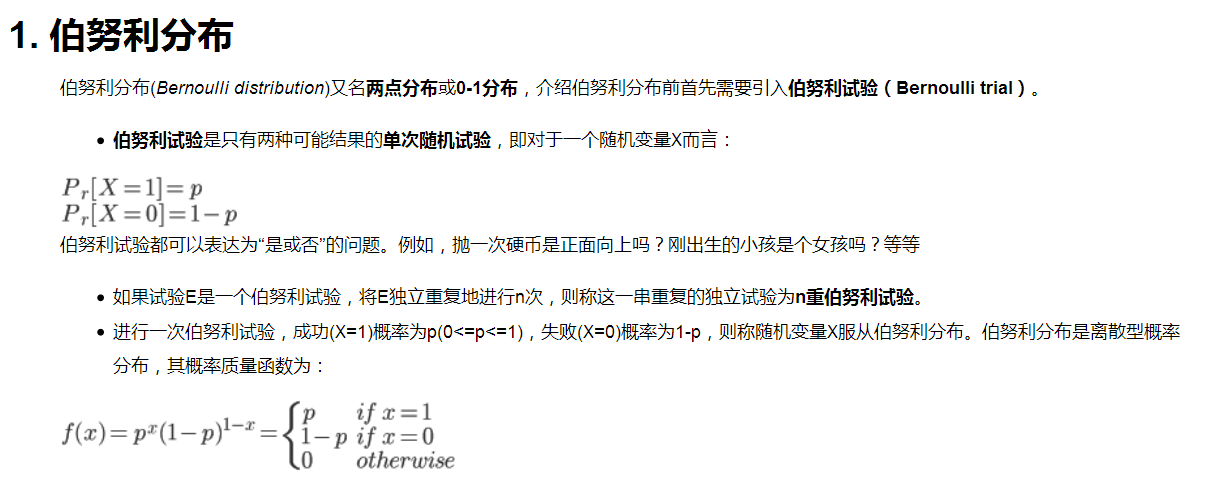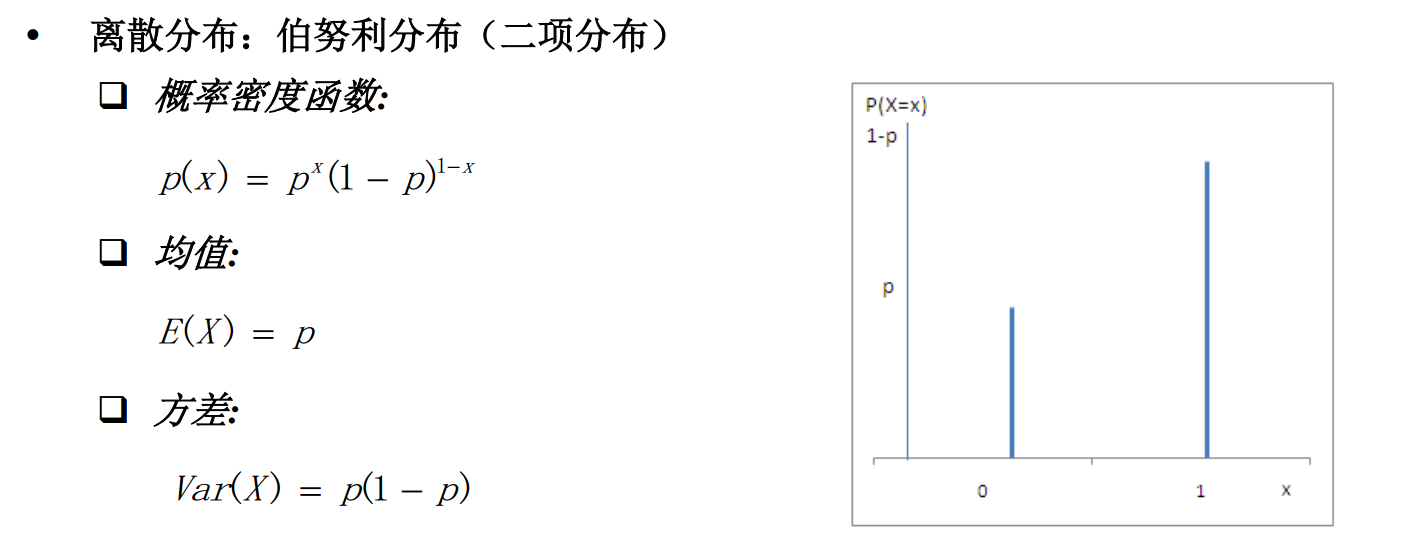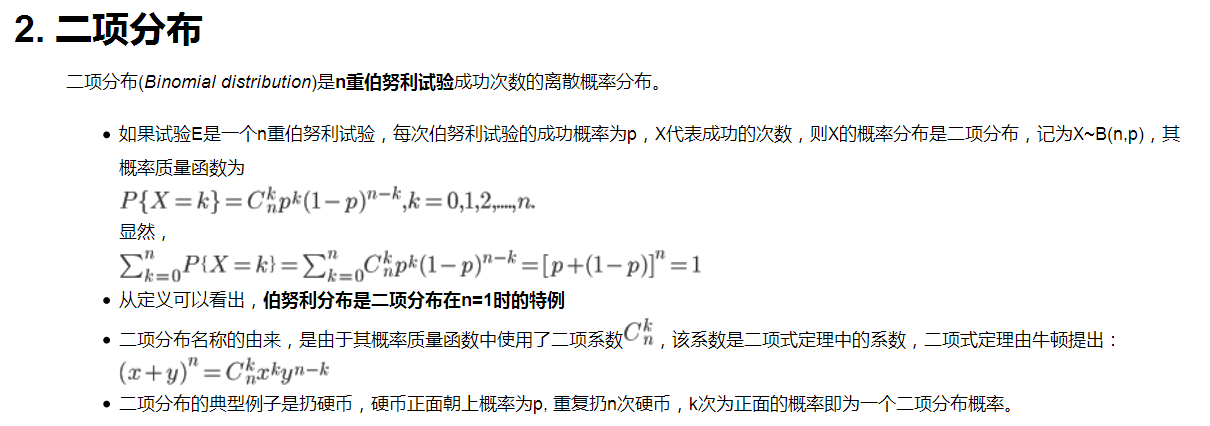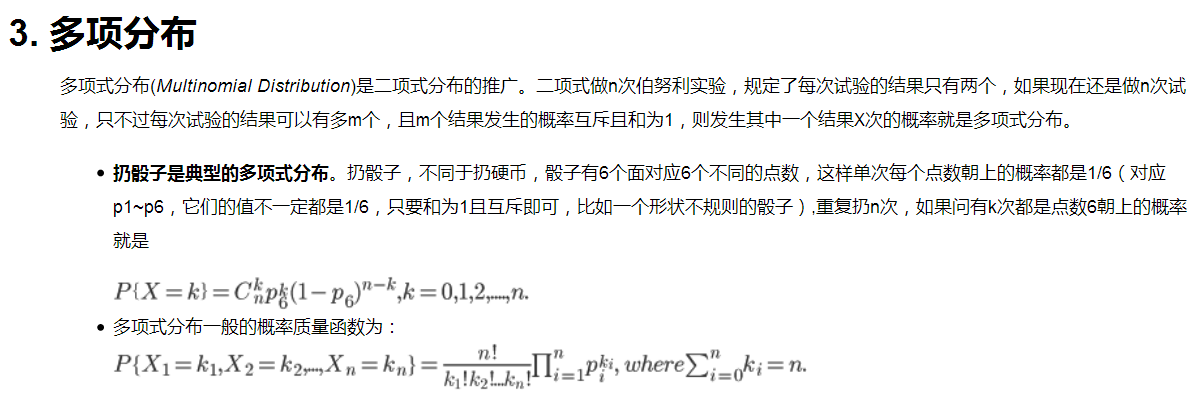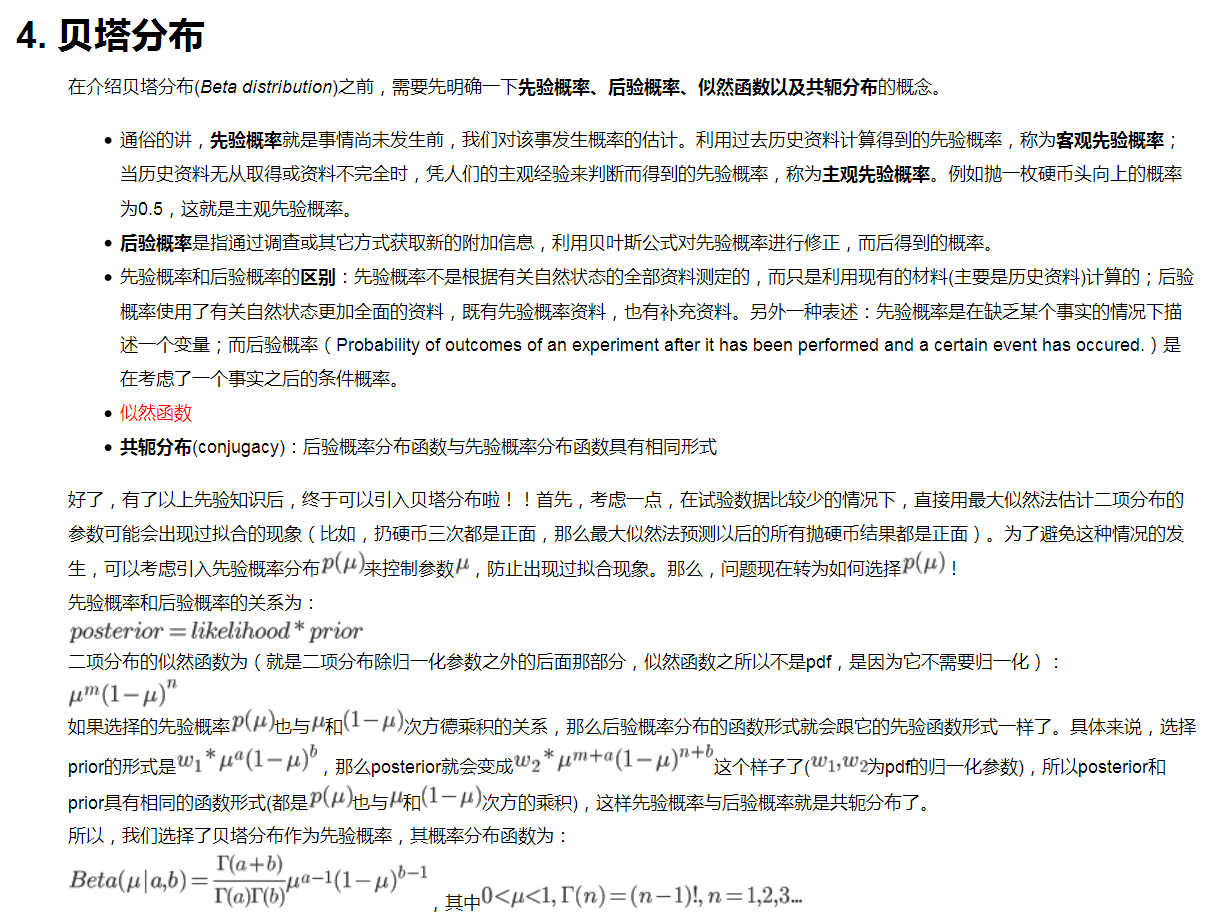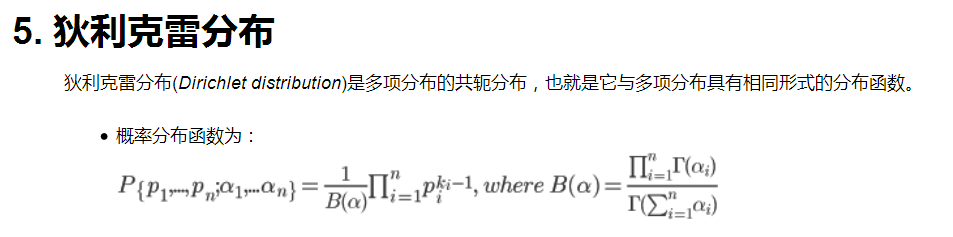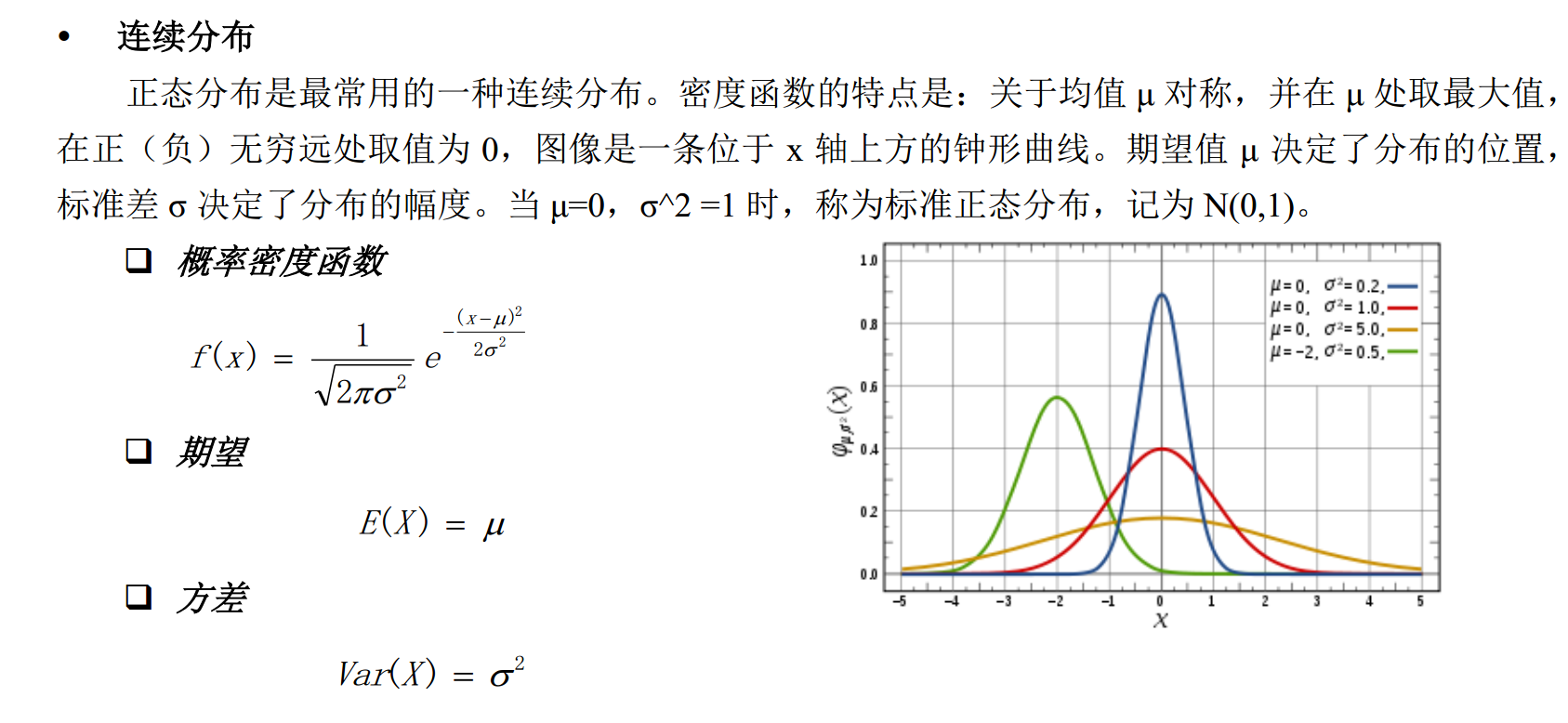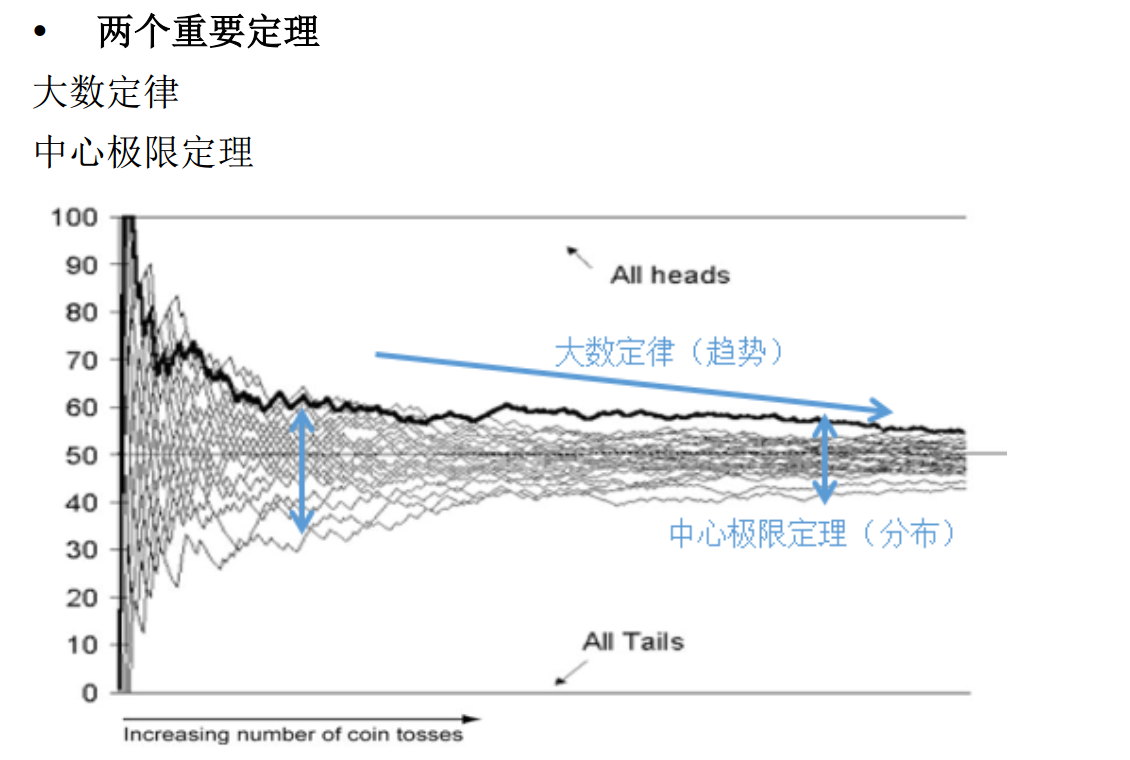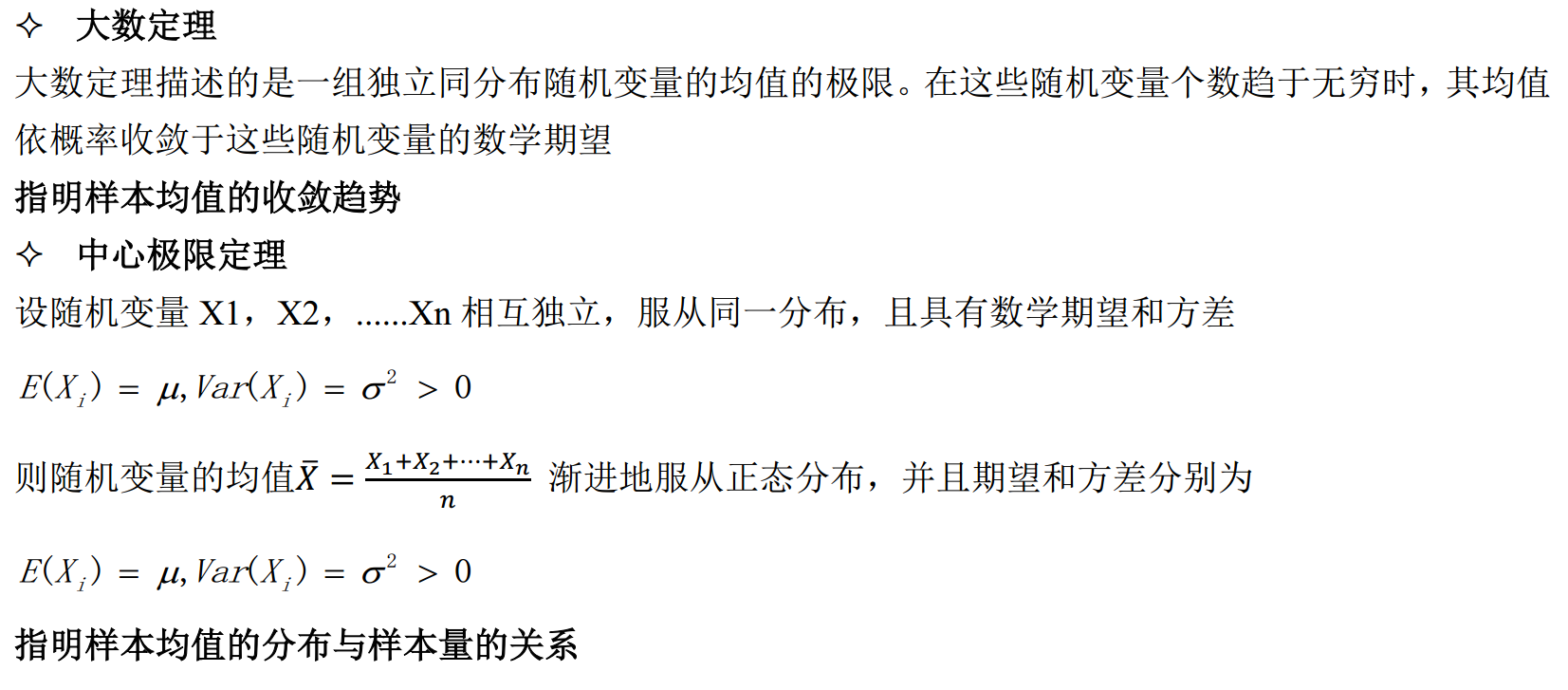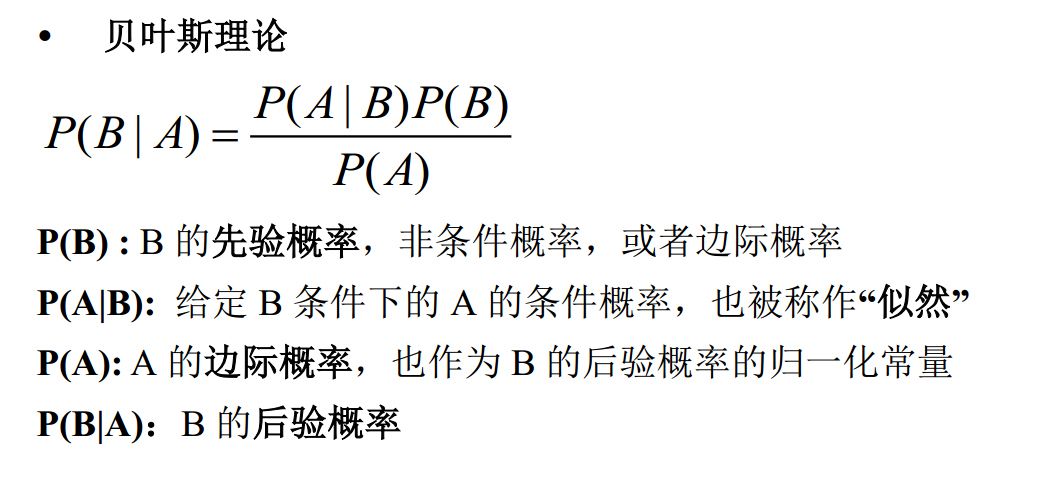展开全文机器学习 伯努利分布 二项分布 贝叶斯理论
• 引入亚纯函数中的对数级概念,讨论半平面上零级Dirichlet级数以及随机Dirichlet级数的增长性,得到了Dirichle级数的对数增长性与其系数之间有关联的几个定理
• Dirichlet's Theorem on Arithmetic Progressions Description If a and d are relatively prime positive integers, the arithmetic sequence beginning with a and increasing by d, i.e., a, a + d, a + 2d,
Dirichlet's Theorem on Arithmetic Progressions
Description
If a and d are relatively prime positive integers, the arithmetic sequence beginning with a and increasing by d, i.e., a, a + d, a + 2d, a + 3d, a + 4d, ..., contains infinitely many prime numbers. This fact is known as Dirichlet's Theorem on Arithmetic Progressions,
which had been conjectured by Johann Carl Friedrich Gauss (1777 - 1855) and was proved by Johann Peter Gustav Lejeune Dirichlet (1805 - 1859) in 1837.
For example, the arithmetic sequence beginning with 2 and increasing by 3, i.e.,
2, 5, 8, 11, 14, 17, 20, 23, 26, 29, 32, 35, 38, 41, 44, 47, 50, 53, 56, 59, 62, 65, 68, 71, 74, 77, 80, 83, 86, 89, 92, 95, 98, ... ,
contains infinitely many prime numbers
2, 5, 11, 17, 23, 29, 41, 47, 53, 59, 71, 83, 89, ... .
Your mission, should you decide to accept it, is to write a program to find the nth prime number in this arithmetic sequence for given positive integers a, d, and n.
Input
The input is a sequence of datasets. A dataset is a line containing three positive integers a, d, and n separated by a space. a and d are relatively prime. You may assume a <= 9307, d <= 346, and n <= 210.
The end of the input is indicated by a line containing three zeros separated by a space. It is not a dataset.
Output
The output should be composed of as many lines as the number of the input datasets. Each line should contain a single integer and should never contain extra characters.
The output integer corresponding to a dataset a, d, n should be the nth prime number among those contained in the arithmetic sequence beginning with a and increasing by d.
FYI, it is known that the result is always less than 106 (one million) under this input condition.
Sample Input
367 186 151
179 10 203
271 37 39
103 230 1
27 104 185
253 50 85
1 1 1
9075 337 210
307 24 79
331 221 177
259 170 40
269 58 102
0 0 0

Sample Output
92809
6709
12037
103
93523
14503
2
899429
5107
412717
22699
25673

分析：最开始的代码貌似会超时，另一个能过，WTF？
code1:
#include<iostream>
#include<cmath>
#include<cstring>
#include<algorithm>
#include<cstdio>
using namespace std;
bool IsPrime(int n)
{
for(int i=2;i<=sqrt(n);i++)
if(n%i==0) return false;
return true;
}
int main()
{
int a,d,n,i,j;
while(scanf("%d %d %d",&a,&d,&n),a&&d&&n)
{
for(i=0,j=0;i!=n;j++)
if(IsPrime(a+j*d))
i++;
if(j==1) printf("%d\n",a+d);
else printf("%d\n",a+(j-1)*d);
}
return 0;
}
code2:
#include<iostream>
using namespace std;
bool judge_prime(int temp)
{
int k,flag=1;
int num=2;
if(temp==2)
return true;
else if(temp==1||temp%2==0)
return false;
else
{
for(k=3;k*k<=temp;k+=2)
if(temp%k==0)
{
flag=0;
break;
}
}
if(flag)
return true;
else
return false;
}
int main(void)
{
int a,b,n,i,temp;
for(;;)
{
int flag=0;
cin>>a>>b>>n;
if(b==0&&n==0||a==0||a>9307||b>346||n>210)
break;
if(b==0&&judge_prime(a)==false)
break;
for(i=0;flag!=n;i++)
{
temp=a+i*b;
if(judge_prime(temp))
flag++;
}
cout<<temp<<endl;
}
return 0;
}
展开全文dataset input integer output
• 作为LDA的预备知识,Dirichlet Multinomial共轭结构很重要,在介绍这个共轭结构之前,先介绍一下将会用到的相关概念 一.Gamma 函数 Gamma函数定义: 分部积分后可得: 不断展开等式右边,进而有: Bohr-...
Welcome To My Blog
作为LDA的预备知识,Dirichlet Multinomial共轭结构很重要,在介绍这个共轭结构之前,先介绍一下将会用到的相关概念

一.Gamma 函数

Gamma函数定义:分部积分后可得:不断展开等式右边,进而有:Bohr-Mullerup定理:
如果f:(0,∞)→(0,∞),且满足:f(1)=1;f(x+1)=xf(x);log(f(x))是凸函数,那么唯一满足以上条件的就是Γ(x)
Gamma函数图像(from Wikipedia):复平面上的Gamma函数(from Wikipedia):如何下函数被称为Digamma函数:这是个很重要的函数,在求Dirichlet分布相关的参数的极大似然估计时往往用到该函数
Digamma函数具有如下性质二.Beta Binomial共轭

在贝叶斯统计中，如果后验分布与先验分布属于同分布，则先验分布与后验分布被称为共轭分布，而先验分布被称为似然函数的共轭先验.
Beta分布是Binomial分布的共轭先验

Beta分布可以通过推导n个独立产生于同一个均匀分布的数字中第k大数字的过程推导出Beta分布,具体可参考靳志辉老师的LDA数学八卦
Beta分布的期望:Beta分布(from Wikipedia)
PDF:probability density function(概率密度函数)因为Beta分布可以拟合多种曲线,所以被广泛使用

二项分布

n是总的试验次数,p是实验成功的概率,k是实验成功的次数
Probability mass function:Beta Binomial共轭

按照贝叶斯推理的过程引出Beta-Binomial共轭:
1. p是要猜的参数,假设p的先验分布为Beta分布,即2. 现有m个数字,知道这m个数字与p的大小关系,其中有m1个数字比p小,m2个数字比p大(m1+m2=m).可知这m个数字与p的大小关系是二项分布(Binomial Distribution)的一个观察值
3. 那么根据m1和m2这个经验,我们便可以得到p的后验分布(证明过程可参考靳志辉老师的LDA数学八卦,并不复杂)后验分布和先验分布都是Beta分布,只不过是参数变了,所以Beta分布式二项分布的共轭先验. 实际上,第一步也可以假设p服从其它分布,只不过因为观察值服从二项分布,所以假设p为Beta分布后,p的后验概率也服从Beta分布,方面计算

三.Dirichlet Multinomial共轭

Dirichlet分布Beta分布就是Dirichlet分布的参数n=2时的情况
Dirichlet分布的期望:或者Dirichlet分布(from Wikipedia)
dirichlet-distribution:LogDirichletDensity-alpha_0.3_to_alpha_2.0 在LDA中用的主要是α＜1的对称Dirichlet分布Multinomial 分布

多项分布是二项分布的推广,举例来说,多项分布建模的是这一问题:有一个k个面的骰子,投掷一次结果是第i的面概率是pi,现独立地投掷n次,结果是第i个面的有xi次,多项分布就是给出了投掷n次后各种结果的概率公式
Probability mass function:Binomial分布就是Multinomial分布的n=2时的情况

Dirichlet Multinomial共轭

类似Beta Binomial共轭的贝叶斯推理:
1. (p1,p2,…pn)是要猜的参数,假设(p1,p2,…pn)的先验分布为Dirichlet分布
2. 现有n个数字(x1,x2,…,xn),知道这n个数字与(p1,p2,…pn)的大小关系,其中有c1个数字比p1小,c2个数字比p1大同时比p2小,cn个数字比p_(n-1)大同时比pn小.这n个数字与(p1,p2,…pn)的大小关系是多项分布的一个观察值
3. 根据(c1,c2,…,cn)这个经验,可以得到(p1,p2,…pn)的后验分布参考:靳志辉,LDA数学八卦
展开全文数学
• 利用中心极限定理和Slutsky定理, 证明了一类Dirichlet 分布的渐近分布为正态分布.
• 例如，它包含AdS解决方案并遵循g定理。 此外，它可以产生一个单点函数，边界Weyl异常及其之间的通用关系的正确表达式。 我们还研究了规范场的相对边界条件，这与重力场的Dirichlet边界条件相对应。 有趣的是，带...
• 讨论了根据给定的双调和函数可以确定一个双解析函数的重要性质...还讨论了双调和函数的Dirichlet问题和变形的Dirichlet问题，并得到了相应的可解性定理。对于双解析函数的Dirichlet问题也得到了相应的可解性结论。
• 是文本语义分析中比较重要的一个模型，同时，LDA模型中使用到了贝叶斯思维的一些知识，这些知识是统计机器学习的基础，这些知识点包括Gamma函数和分布，Beta函数和分布，Dirichlet函数和分布，贝叶斯定理，Gibbs采样...
引言

LDA(Latent Dirichlet Allocation)称为潜在狄利克雷分布，是文本语义分析中比较重要的一个模型，同时，LDA模型中使用到了贝叶斯思维的一些知识，这些知识是统计机器学习的基础。为了能够对LDA原理有清晰的认识，也为了能够对贝叶斯思维有全面的了解，在这里对基本知识以及LDA的相关知识进行阐述，本系列包括两个部分：

Latent Dirichlet Allocation——理论篇
Latent Dirichlet Allocation——实践篇

在理论篇中将重点阐述贝叶斯相关的知识和LDA的基本思想，基本的知识点包括Gamma函数和分布，Beta函数和分布，Dirichlet函数和分布，贝叶斯定理，Gibbs采样等等。在接下来的文章，我们通过以下几个方面具体介绍LDA的核心思想：

基础知识：二项分布，多项式分布，Gamma分布，Beta分布，Dirichlet分布，贝叶斯定理，共轭分布
文本建模：Unigram Model，概率主题模型，Gibbs采样以及贝叶斯推理

一、基础知识

在贝叶斯思维以及LDA中需要使用到一些概率的知识，下面我们罗列下会使用到的一些基本知识。

1、二项分布

二项分布是概率分布里面最简单也是最基本的分布，要理解二项分布，我们首先得定义n$n$次独立重复试验的概念：n$n$次独立重复试验是指在相同的条件下，重复做的n$n$次试验称为n$n$次独立重复试验。

假设对于一个事件A$A$，在一次试验中，其发生的概率为p$p$，那么其不发生的概率为1−p$1-p$，那么在n$n$次独立重复试验中事件A$A$恰好发生k$k$次的概率为：

Pn(k)=Cknpk(1−p)n−kP_n\left ( k \right )=C_n^kp^{k}\left ( 1-p \right )^{n-k}

在这里，参数k$k$是一个随机变量，便称这样的随机变量k$k$服从二项分布，记为：k∼B(n,p)$k\sim B\left ( n,p \right )$。

可以验证下式成立：

∑k=0nPn(k)=∑k=0nCknpk(1−p)n−k=1\sum _{k=0}^{n}P_n\left ( k \right )=\sum _{k=0}^{n}C_n^kp^{k}\left ( 1-p \right )^{n-k}=1

2、多项式分布

多项式分布是二项分布的一个推广形式，在二项分布中，事件A$A$的取值可能只能是发生或者是没有发生，而在多项式分布中事件A$A$的取值可能有k$k$种，取每一种可能的概率为pi$p_i$，其中pi$p_i$满足：

∑i=1kpi=1\sum _{i=1}^{k}p_i=1

多项式分布的概率形式为：

P(x1,x2,⋯,xk;n;p1,p2,⋯,pk)=n!x1!x2!⋯xk!px11px22⋯pxkkP\left ( x_1,x_2,\cdots ,x_k\; ;n\; ;p_1,p_2,\cdots ,p_k \right )=\frac{n!}{x_1!x_2!\cdots x_k!}p_1^{x_1}p_2^{x_2}\cdots p_k^{x_k}

3、Gamma分布

Gamma函数的具体形式如下：

Γ(x)=∫∞0e−uux−1du\Gamma \left ( x \right )=\int_{0}^{\infty }e^{-u}u^{x-1}du

其中，x>0$x> 0$。Gamma函数的图像如下所示：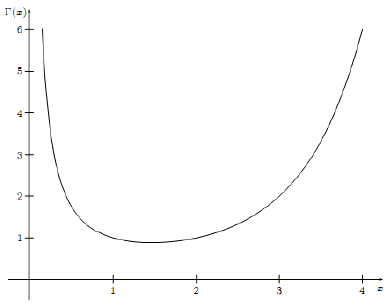Gamma函数Γ(x)$\Gamma \left ( x \right )$具有如下的一些性质：

性质1：

Γ(x+1)=xΓ(x)\Gamma \left ( x+1 \right )=x\Gamma \left ( x \right )

这个性质可以通过分部积分的方法得到证明，证明如下：

Γ(x+1)=∫∞0e−uuxdu=∫∞0−uxde−u=−uxe−u∣∞0−∫∞0−euxux−1du=x∫∞0e−uux−1du=xΓ(x)\begin{matrix}
\Gamma \left ( x+1 \right )=\int_{0}^{\infty }e^{-u}u^{x}du\\
=\int_{0}^{\infty }-u^{x}de^{-u}=-u^xe^{-u}\mid _0^{\infty }-\int_{0}^{\infty }-e^{u}xu^{x-1}du\\
=x\int_{0}^{\infty }e^{-u}u^{x-1}du=x\Gamma \left ( x \right )
\end{matrix}

性质2：

Γ(1)=1,Γ(12)=π√\Gamma \left ( 1 \right )=1,\; \Gamma \left ( \frac{1}{2} \right )=\sqrt{\pi }

性质3：

Γ(n+1)=n!\Gamma \left ( n+1 \right )=n!

4、Beta分布

Beta函数的具体形式如下：

Beta(a,b)=∫10xa−1(1−x)b−1dxBeta\left ( a,b \right )=\int_{0}^{1}x^{a-1}\left ( 1-x \right )^{b-1}dx

其中，a>0,b>0$a> 0,\; b>0$。Beta函数有如下的一个性质：

Beta(a,b)=Γ(a)Γ(b)Γ(a+b)Beta\left ( a,b \right )=\frac{\Gamma \left ( a \right )\Gamma \left ( b \right )}{\Gamma \left ( a+b \right )}

上述的关于Beta函数的性质将Beta函数与Gamma函数联系起来，对于该性质的证明如下所示：

Γ(a)Γ(b)=∫∞0e−uua−1du⋅∫∞0e−vvb−1dv=∫∞0∫∞0e−(u+v)ua−1vb−1dudv\begin{matrix}
\Gamma \left ( a \right )\Gamma \left ( b \right )=\int_{0}^{\infty }e^{-u}u^{a-1}du\cdot \int_{0}^{\infty }e^{-v}v^{b-1}dv\\
=\int_{0}^{\infty }\int_{0}^{\infty }e^{-\left ( u+v \right )}u^{a-1}v^{b-1}dudv\\
\end{matrix}

此时，令z=u+v$z=u+v$，t=uu+v$t=\frac{u}{u+v}$，上式可转化为：

Γ(a)Γ(b)=∫∞0∫10e−z(zt)a−1[z(1−t)]b−1zdzdt=∫∞0e−zza−1zdz⋅∫10ta−1(1−t)b−1dt=Γ(a+b)⋅∫10ta−1(1−t)b−1dt=Γ(a+b)⋅Beta(a,b)\begin{matrix}
\Gamma \left ( a \right )\Gamma \left ( b \right )=\int_{0}^{\infty }\int_{0}^{1}e^{-z}\left ( zt \right )^{a-1}\left [ z\left ( 1-t \right ) \right ]^{b-1}zdzdt\\
=\int_{0}^{\infty }e^{-z}z^{a-1}zdz\cdot \int_{0}^{1}t^{a-1}\left ( 1-t \right )^{b-1}dt\\
=\Gamma \left ( a+b \right )\cdot \int_{0}^{1}t^{a-1}\left ( 1-t \right )^{b-1}dt\\
=\Gamma \left ( a+b \right )\cdot Beta\left ( a,b \right )
\end{matrix}

由此可知：Beta(a,b)=Γ(a)Γ(b)Γ(a+b)$Beta\left ( a,b \right )=\frac{\Gamma \left ( a \right )\Gamma \left ( b \right )}{\Gamma \left ( a+b \right )}$。

5、Dirichlet分布

Dirichlet函数的基本形式为：

D(a1,a2,⋯,ak)=∫⋯∫xa1−11⋯xak−1kdx1⋯dxkD\left ( a_1,a_2,\cdots ,a_k \right )=\int \cdots \int x_1^{a_1-1}\cdots x_k^{a_k-1}dx_1\cdots dx_k

其中，x1⋯xk⩾0$x_1\cdots x_k\geqslant 0$，∑ki=1xi=1$\sum _{i=1}^{k}x_i=1$。而Dirichlet分布的概率密度函数为：

p(x1,⋯,xk)=1D(a1,⋯,ak)xa11⋯xakkp\left ( x_1,\cdots ,x_k \right )=\frac{1}{D\left ( a_1,\cdots ,a_k \right )}x_1^{a_1}\cdots x_k^{a_k}

其中，0⩽x1⋯xn⩽1$0\leqslant x_1\cdots x_n\leqslant 1$，且∑ki=1xk=1$\sum_{i=1}^{k}x_k=1$，D(a1,⋯,ak)$D\left ( a_1,\cdots ,a_k \right )$的形式为：

D(a1,⋯,ak)=Γ(a1)⋯Γ(ak)Γ(a1+⋯+ak)D\left ( a_1,\cdots ,a_k \right )=\frac{\Gamma \left ( a_1 \right )\cdots \Gamma \left ( a_k \right )}{\Gamma \left ( a_1+\cdots +a_k \right )}

注意到Beta分布是特殊的Dirichlet分布，即k=2$k=2$时的Dirichlet分布。

6、贝叶斯定理

贝叶斯定理中牵涉到概率的一些基本知识，包括：

条件概率
联合概率
边缘概率

条件概率的表达形式为：P(A∣B)$P\left ( A\mid B \right )$，其表示的含义是事件A$A$在事件B$B$发生的条件下发生的概率。

联合概率的表达形式为：P(A,B)$P\left ( A,\; B \right )$，其表示的含义是事件A$A$和事件B$B$同时发生的概率。

事件A$A$的边缘概率的表达形式为：P(A)$P\left ( A \right )$，其表示的含义是事件A$A$发生的概率。

有了以上的定义，贝叶斯定理可以通过如下的贝叶斯公式表示：

P(B∣A)=P(A∣B)P(B)P(A)P\left ( B\mid A \right )=\frac{P\left ( A\mid B \right )P\left ( B \right )}{P\left ( A \right )}

对于上述的贝叶斯公式，P(B)$P\left ( B \right )$称为先验概率，即在得到新的数据前某一假设的概率；P(B∣A)$P\left ( B\mid A \right )$称为后验概率，即在得到了新的数据后，对原假设的修正；P(A)$P\left ( A \right )$称为标准化常量；P(A∣B)$P\left ( A\mid B \right )$称为似然度。

对于两个相互独立的事件的联合概率有如下的性质：

P(A,B)=P(A)P(B)P\left ( A,\; B \right )=P\left ( A \right )P\left ( B \right )

7、共轭分布

有了如上的贝叶斯定理，对于贝叶斯派而言，有如下的思考方式：

先验分布+样本信息⇒$\Rightarrow$后验分布

上述的形式定义是贝叶斯派的思维方式，人们对于事物都会存在着最初的认识（先验分布），随着收集到越来越多的样本信息，新观察到的样本信息会不断修正人们对事物的最初的认识，最终得到对事物较为正确的认识（后验分布）。若这样的后验概率P(θ∣x)$P\left ( \theta \mid x \right )$和先验概率P(x)$P\left ( x \right )$满足同样的分布，那么先验分布和后验分布被称为共轭分布，同时，先验分布叫做似然函数的共轭先验分布。

有了如上的的共轭先验分布的定义，有如下的两个性质：

1、Beta分布是二项分布的共轭先验分布，即：
Beta(p∣α,β)+Count(m1,m2)=Beta(p∣α+m1,β+m2)Beta\left ( p\mid \alpha ,\beta  \right )+Count\left ( m_1,m_2 \right )=Beta\left ( p\mid \alpha +m_1 ,\beta +m_2 \right )

对于上式，对于事件A$A$，假设其发生的概率为p$p$，不发生的概率为1−p$1-p$，发生的次数为m1$m_1$，不发生的概率为m2$m_2$，且有m1+m2=n$m_1+m_2=n$。则对于参数m1$m_1$，则有：

P(m1∣p)=pm1(1−p)n−m1=pm1(1−p)m2P\left ( m_1\mid p \right )=p^{m_1}\left ( 1-p \right )^{n-m_1}=p^{m_1}\left ( 1-p \right )^{m_2}

而对于参数p$p$，则是服从参数为α$\alpha$和β$\beta$的Beta分布：

P(p∣α,β)=pa−1(1−p)b−1∫10pa−1(1−p)b−1dpP\left ( p\mid \alpha ,\beta \right )=\frac{p^{a-1}\left ( 1-p \right )^{b-1}}{\int_{0}^{1}p^{a-1}\left ( 1-p \right )^{b-1}dp}

已知在贝叶斯定理中有如下的公式成立：

P(B∣A)=P(A∣B)P(B)P(A)∝P(A∣B)P(B)P\left ( B\mid A \right )=\frac{P\left ( A\mid B \right )P\left ( B \right )}{P\left ( A \right )}\propto P\left ( A\mid B \right )P\left ( B \right )

则对于上述的后验概率，即为：

P(p∣m1)=P(m1∣p)⋅P(p)P(m1)∝P(m1∣p)⋅P(p)=pm1(1−p)m2⋅pa−1(1−p)b−1∫10pa−1(1−p)b−1dp∝pm1(1−p)m2⋅pa−1(1−p)b−1=pa+m1−1(1−p)b+m2−1\begin{matrix}
P\left ( p\mid m_1 \right )=\frac{P\left ( m_1\mid p \right )\cdot P\left ( p \right )}{P\left ( m_1 \right )}\\
\propto P\left ( m_1\mid p \right )\cdot P\left ( p \right )\\
=p^{m_1}\left ( 1-p \right )^{m_2}\cdot \frac{p^{a-1}\left ( 1-p \right )^{b-1}}{\int_{0}^{1}p^{a-1}\left ( 1-p \right )^{b-1}dp}\\
\propto p^{m_1}\left ( 1-p \right )^{m_2}\cdot p^{a-1}\left ( 1-p \right )^{b-1}\\
=p^{a+m_1-1}\left ( 1-p \right )^{b+m_2-1}
\end{matrix}

由上可知，Beta分布是二项分布的共轭先验分布。

2、Dirichlet分布是多项式分布的共轭先验分布，即：
Dir(p⃗ ∣α⃗ )+MultCount(m⃗ )=Dir(p⃗ ∣α⃗ +m⃗ )Dir\left ( \vec{p}\mid \vec{\alpha } \right )+MultCount\left ( \vec{m} \right )=Dir\left ( \vec{p}\mid \vec{\alpha }+\vec{m} \right )

我们对上式采用与Beta分布同样的证明方式，对于多项式分布，有下式成立：

P(m⃗ ∣p⃗ )=pm11pm22⋯pmkkP\left ( \vec{m}\mid \vec{p} \right )=p_1^{m_1}p_2^{m_2}\cdots p_k^{m_k}

然而概率p⃗ $\vec{p}$服从的参数为α⃗ $\vec{\alpha }$的Dirichlet分布，即：

P(p⃗ ∣α⃗ )=pα11pα22⋯pαkkD(α1,α2,⋯,αk)P\left ( \vec{p}\mid \vec{\alpha } \right )=\frac{p_1^{\alpha _1}p_2^{\alpha _2}\cdots p_k^{\alpha _k}}{D\left ( \alpha _1,\alpha _2,\cdots ,\alpha _k \right )}

由贝叶斯定理可知：

P(p⃗ ∣m⃗ )=P(m⃗ ∣p⃗ )⋅P(p⃗ )P(m⃗ )∝P(m⃗ ∣p⃗ )⋅P(p⃗ )=pm11pm22⋯pmkk⋅pα11pα22⋯pαkkD(α1,α2,⋯,αk)∝pm11pm22⋯pmkk⋅pα11pα22⋯pαkk=pm1+α11pm2+α22⋯pmk+αkk\begin{matrix}
P\left ( \vec{p}\mid \vec{m} \right )=\frac{P\left ( \vec{m}\mid \vec{p} \right )\cdot P\left ( \vec{p} \right )}{P\left ( \vec{m} \right )}\\
\propto P\left ( \vec{m}\mid \vec{p} \right )\cdot P\left ( \vec{p} \right )\\
=p_1^{m_1}p_2^{m_2}\cdots p_k^{m_k}\cdot \frac{p_1^{\alpha _1}p_2^{\alpha _2}\cdots p_k^{\alpha _k}}{D\left ( \alpha _1,\alpha _2,\cdots ,\alpha _k \right )}\\
\propto p_1^{m_1}p_2^{m_2}\cdots p_k^{m_k}\cdot p_1^{\alpha _1}p_2^{\alpha _2}\cdots p_k^{\alpha _k}\\
=p_1^{m_1+\alpha _1}p_2^{m_2+\alpha _2}\cdots p_k^{m_k+\alpha _k}
\end{matrix}

由此可知，Dirichlet分布是多项式分布的共轭先验分布。

二、文本建模

对于一篇文章，是文章中出现的次的过程，在文章中，我们已经知道每个词出现的概率，则在省城文章的过程中，我们在词库中根据概率取出每个词，形成一篇文章。

1、Unigram Model

1.1、频率派

上述的过程说明了最简单的文本是如何产生的，我们对上述的过程数学化，假设：

词库中(即对所有文档中的词去停用词)共有V$V$个词：v1,v2,⋯,vV$v_1,v_2,\cdots ,v_V$；
词库中每一个词出现的次数记为：n1,n2,⋯,nV$n_1,n_2,\cdots ,n_V$，所有词出现的总次数为N$N$；
每个词对用的概率记为：p⃗ ={p1,p2,⋯,pV}$\vec{p}=\left \{ p_1,p_2,\cdots ,p_V \right \}$。

假设有m$m$篇文档，记为W=(w⃗ 1,w⃗ 2,⋯,w⃗ m)$W=\left ( \vec{w}_1,\vec{w}_2,\cdots ,\vec{w}_m \right )$，则整个文档的概率为：

P(W)=P(w⃗ 1,w⃗ 2,⋯,w⃗ m)P\left ( W \right )=P\left ( \vec{w}_1,\vec{w}_2,\cdots ,\vec{w}_m \right )

在这里，我们假设文档与文档之间是相互独立的，而且进一步词与词之间也是相互独立的——词袋模型(Bag-of-words)。词袋模型表名词的顺序是无关紧要。基于这样的假设后上述的概率可以表示为：

P(W)=P(w⃗ 1)P(w⃗ 2)⋯P(w⃗ m)P\left ( W \right )=P\left ( \vec{w}_1 \right )P\left ( \vec{w}_2 \right )\cdots P\left ( \vec{w}_m \right )

对所有的这m$m$篇文档中，词vi$v_i$出现的次数为ni$n_i$，由于文档与文档之间是相互独立的，且词与词之间也是相互独立的，则对于所有的文档集W$W$，我们将相同的词记在一起，即对于词vi$v_i$，记为总共出现的次数为ni$n_i$。从词库中选择每个词的过程满足多项式分布，总共需要从词库中抽取N$N$次，则全体文档的概率为：

P(W)=P(w⃗ 1)P(w⃗ 2)⋯P(w⃗ m)=∏k=1N∏i=1Vpnii\begin{matrix}
P\left ( W \right )=P\left ( \vec{w}_1 \right )P\left ( \vec{w}_2 \right )\cdots P\left ( \vec{w}_m \right )\\
=\prod_{k=1}^{N}\prod_{i=1}^{V}p_i^{n_i}
\end{matrix}

至此，已经计算出全部文档的联合概率，但是对于每个词被选择的概率pi$p_i$是一个未知数，一个很重要的任务就是估计上式中的每个词被选择的概率，通常使用的方法是使用最大似然估计的方法：

取上式的log似然函数：

log(P(W))=N⋅∑i=1Vni⋅log(pi)log\; \left ( P\left ( W \right ) \right )=N\cdot \sum_{i=1}^{V}n_i\cdot log\left ( p_i \right )

对上述似然函数取最大值，即对每个概率值pi$p_i$求导数：

最终，可以求得参数pi$p_i$的估计值：

pi=niNp_i=\frac{n_i}{N}

1.2、贝叶斯派

对于贝叶斯派来说，其并不认同上述的求解参数值估计的方法，贝叶斯思维认为，一切的参数都是随机变量，因此上述的选择每个词的概率不是一个确定的值，而是一个随机变量，随机变量就应该服从一个分布。因此参数p⃗ $\vec{p}$是由分布P(p⃗ )$P\left ( \vec{p} \right )$决定的，该分布称为先验分布。则上述的过程就变成了如下的两个过程：

首先由先验分布P(p⃗ )$P\left ( \vec{p} \right )$得到参数的样本p⃗ $\vec{p}$；
由参数p⃗ $\vec{p}$生成文档。

上述的过程，可以由下面的概率图模型表示：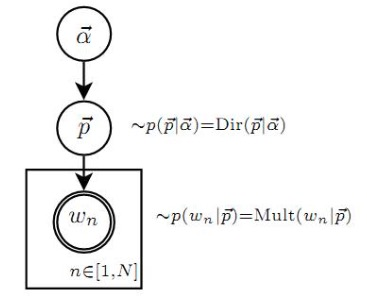依据上述的观点，则文档的概率可以表示为：

P(W)=∫P(W∣p⃗ )⋅P(p⃗ )dp⃗ P\left ( W \right )=\int P\left ( W\mid \vec{p} \right )\cdot P\left ( \vec{p} \right )d\vec{p}

此处的P(p⃗ )$P\left ( \vec{p} \right )$称为先验分布，已知P(W∣p⃗ )$P\left ( W\mid \vec{p} \right )$服从多项式分布，由上述的共轭分布的知识可知：

多项式分布的共轭分布是Dirichlet分布。

因此对于先验分布P(p⃗ )$P\left ( \vec{p} \right )$可以选择为Dirichlet分布：

Dir(p⃗ ∣α⃗ )=1Δ(α⃗ )∏i=1Vpαi−1iDir\left ( \vec{p}\mid \vec{\alpha } \right )=\frac{1}{\Delta \left ( \vec{\alpha } \right )}\prod_{i=1}^{V}p_i^{\alpha _i-1}

其中，α⃗ =(α1,α2,⋯,αV)$\vec{\alpha }=\left ( \alpha _1,\alpha _2,\cdots ,\alpha _V \right )$，Δ(α⃗ )$\Delta \left ( \vec{\alpha } \right )$称为归一化因子：

Δ(α⃗ )=∫∏i=1Vpαi−1idp⃗ \Delta \left ( \vec{\alpha } \right )=\int \prod_{i=1}^{V}p_i^{\alpha _i-1}d\vec{p}

由共轭分布的知识可知：

先验分布为Dirichlet分布+多项分布的数据知识=后验分布为Dirichlet分布
Dir(p⃗ ∣α⃗ )+MultCount(n⃗ )=Dir(p⃗ ∣α⃗ +n⃗ )Dir \left ( \vec{p}\mid \vec{\alpha } \right )+MultCount\left ( \vec{n} \right )=Dir \left ( \vec{p}\mid \vec{\alpha }+\vec{n} \right )

基于上述的共轭分布的性质，已知了参数p⃗ $\vec{p}$的先验分布为Dir(p⃗ ∣α⃗ )$Dir \left ( \vec{p}\mid \vec{\alpha } \right )$，对于每个词出现的次数的统计服从多项式分布，则可以通过上述的性质得到后验分布：

P(p⃗ ∣W,α⃗ )=Dir(p⃗ ∣n⃗ +a⃗ )=1Δ(n⃗ +a⃗ )∏i=1Vpni+αi−1iP\left ( \vec{p}\mid W,\vec{\alpha } \right )=Dir\left ( \vec{p}\mid \vec{n}+\vec{a} \right )\\=\frac{1}{\Delta \left ( \vec{n}+\vec{a} \right )}\prod_{i=1}^{V}p_i^{n_i+\alpha _i-1}

为了求得后验分布中的参数p⃗ $\vec{p}$，可以使用其均值来估计每一个参数，即：

E(p⃗ )=(n1+α1∑Vi=1(ni+αi),n2+α2∑Vi=1(ni+αi),⋯,nV+αV∑Vi=1(ni+αi))E\left ( \vec{p} \right )=\left ( \frac{n_1+\alpha _1}{\sum_{i=1}^{V}\left ( n_i+\alpha _i \right )},\frac{n_2+\alpha _2}{\sum_{i=1}^{V}\left ( n_i+\alpha _i \right )},\cdots , \frac{n_V+\alpha _V}{\sum_{i=1}^{V}\left ( n_i+\alpha _i \right )} \right )

即：

p^i=ni+αi∑Vi=1(ni+αi)\hat{p}_i=\frac{n_i+\alpha _i}{\sum_{i=1}^{V}\left ( n_i+\alpha _i \right )}

对于整个文本的概率：

P(W∣α⃗ )=∫P(W∣p⃗ )⋅P(p⃗ ∣α⃗ )dp⃗ P\left ( W\mid \vec{\alpha } \right )=\int P\left ( W\mid \vec{p} \right )\cdot P\left ( \vec{p}\mid \vec{\alpha } \right )d\vec{p}

由于P(W∣p⃗ )$P\left ( W\mid \vec{p} \right )$服从多项式分布，而P(p⃗ ∣α⃗ )$P\left ( \vec{p}\mid \vec{\alpha } \right )$服从的Dirichlet分布，则上式可以表示成：

P(W∣α⃗ )=∫∏i=1Vpnii⋅Dir(p⃗ ∣α⃗ )dp⃗ =∫∏i=1Vpnii⋅1Δ(α⃗ )∏i=1Vpαi−1idp⃗ =1Δ(α⃗ )∫∏i=1Vpni+αi−1idp⃗ \begin{matrix}
P\left ( W\mid \vec{\alpha } \right )=\int \prod_{i=1}^{V}p_i^{n_i}\cdot Dir\left ( \vec{p}\mid \vec{\alpha } \right )d\vec{p}\\
=\int  \prod_{i=1}^{V}p_i^{n_i}\cdot \frac{1}{\Delta \left ( \vec{\alpha } \right )}\prod_{i=1}^{V}p_i^{\alpha _i-1}d\vec{p}\\
=\frac{1}{\Delta \left ( \vec{\alpha } \right )}\int \prod_{i=1}^{V}p_i^{n_i+\alpha _i-1}d\vec{p}
\end{matrix}

而已知：Δ(α⃗ )=∫∏Vi=1pαi−1idp⃗ $\Delta \left ( \vec{\alpha } \right )=\int \prod_{i=1}^{V}p_i^{\alpha _i-1}d\vec{p}$，则上式可以表示成：

P(W∣α⃗ )=Δ(n⃗ +α⃗ )Δ(α⃗ )P\left ( W\mid \vec{\alpha } \right )=\frac{\Delta \left ( \vec{n}+\vec{\alpha } \right )}{\Delta \left ( \vec{\alpha } \right )}

2、概率主题模型

前面对文档的生成方式做了简单的介绍，其实在写文章的过程中，每一篇文章都会有一些主题，表示这篇文章主要讲的是关于哪方面的文章，如本篇文章主要是在介绍贝叶斯，LDA等等，而文章的基本组成单元式词，文章的主题则主要表现在词在不同组题的分布上，每一个词是在这些确定的主题上产生的，具体的如下图所示：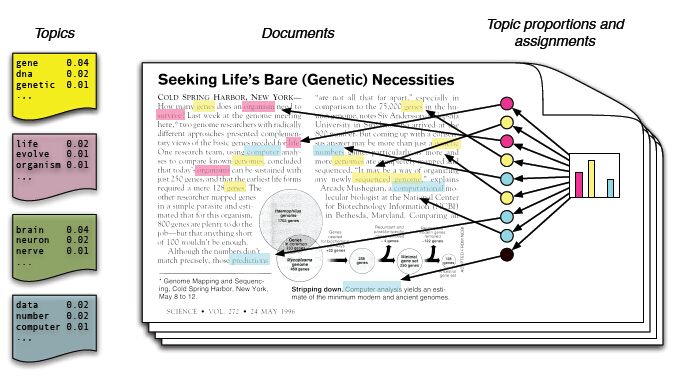文章的主题最终体现在词在每个主题的分布上。在写文章的过程中，首先我们需要做的是确定文章的主题，在确定了文章的主题的前提下，我们产生每一个词，从而构成了整篇文章。

如果要写一篇文章，我们往往是先确定其主题，比如这篇文章是写社会的，还是写的技术类的，或者游记类的，在主题确定的条件下，如要写一篇关于机器学习方面的文章，在确定了主题的条件下，会谈及到损失函数，模型，神经网络，深度学习等等，每个词在这篇文章中的比重会有所不同。这便是文章的生成过程，即：

一篇文章，通常是由多个主题构成的，而每个主题大概可以用于该主题相关的频率最高的一些词来描述。

在上面们提及到一篇文章的生成过程，即：

对于文章选择主题
每个主题下对词汇的选择

2.1、频率派

频率派的观点是选择每个主题的概率和根据主题选择具体词的概率都是具体的值，根据上述的概率主题模型的思想，我们假设文档集中有M$M$篇文档，每一篇文章对应的主题的概率为：θ⃗ m,m∈[1,M]$\vec{\theta} _m, m\in \left [ 1,M \right ]$，在选定了文章的主题后，对于一篇文章，可能会有几个主题，此时，在主题确定的条件下，选择每个主题对应的词，对于第m$m$篇文章中的第n$n$个词，有其所属主题的编号zm,n$z_{m,n}$，假设有K$K$个主题，在每个主题下选择词的概率为：φ⃗ 1,φ⃗ 2,⋯,φ⃗ K$\vec{\varphi} _1,\vec{\varphi} _2,\cdots ,\vec{\varphi} _K$，在对应的第k$k$个主题下依据概率φ⃗ k$\vec{\varphi }_k$选择词。

注意：这里的文档与文档之间是相互独立的，同一个文档中的词与词之间也是相互独立的。

因此，上述过程中很多步骤是可以合并在一起的，同样，我们有如下的假设：

词库中(即对所有文档中的词去停用词)共有V$V$个词：v1,v2,⋯,vV$v_1,v_2,\cdots ,v_V$；
词库中每一个词出现的次数记为：n1,n2,⋯,nV$n_1,n_2,\cdots ,n_V$，所有词出现的总次数为N$N$；
第k$k$个主题下对应的词的概率为：φ⃗ k={φk,1,φk,2,⋯,φk,V}$\vec{\varphi }_k=\left \{ \varphi _{k,1},\varphi _{k,2},\cdots ,\varphi _{k,V} \right \}$，其中k∈[1,K]$k\in \left [ 1,K \right ]$；
第m$m$篇文档对应的主题的概率记为：θ⃗ m={θm,1,θm,2,⋯,θm,K}$\vec{\theta }_m=\left \{ \theta _{m,1},\theta _{m,2},\cdots ,\theta _{m,K} \right \}$，其中m∈[1,M]$m\in \left [ 1,M \right ]$；
对于每一篇文章中对应的词所属主题的编号为：zm,n$z_{m,n}$。

则对于第m$m$篇文档dm$d_m$中的每一个词的生成概率为：

P(w∣dm)=∑z=1KP(w∣z)P(z∣dm)P\left ( w\mid d_m \right )=\sum_{z=1}^{K}P\left ( w\mid z \right )P\left ( z\mid d_m \right )

其中P(z∣dm)$P\left ( z\mid d_m \right )$表示的是在每一篇文章对应的主题的编号，可以由θm,z$\theta _{m,z}$表示；P(w∣z)$P\left ( w\mid z \right )$表示的是在主题编号确定的条件下选择词的概率，可以由φz,w$\varphi _{z,w}$，因此上式可以表示成：

P(w∣dm)=∑z=1Kφz,w⋅θm,zP\left ( w\mid d_m \right )=\sum_{z=1}^{K}\varphi _{z,w}\cdot \theta _{m,z}

由于在文档中词与词之间是相互独立的，因此对于一篇文档，其生成概率为：

P(w⃗ ∣dm)=∏i=1Nm∑z=1KP(wi∣z)P(z∣dm)=∏i=1Nm∑z=1Kφz,wi⋅θm,z\begin{matrix}
P\left ( \vec{w}\mid d_m \right )=\prod_{i=1}^{N_m}\sum_{z=1}^{K}P\left ( w_i\mid z \right )P\left ( z\mid d_m \right )\\
=\prod_{i=1}^{N_m}\sum_{z=1}^{K}\varphi _{z,w_i}\cdot \theta _{m,z}
\end{matrix}

2.2、贝叶斯派

上面介绍的思路中，对于文档选择主题的概率以及依据主题选择每一个词的概率都是固定的数，对于贝叶斯派来说，这是无法接受的，贝叶斯派认为所有的值都是随机变量，因此，在文档对应的主题以及依据指定的主题选择每一个词的概率都服从特定的分布。因此上述的过程可以通过如下的概率图模型表示：该图可以分解成如下的两个部分：

1、α⃗ →θ⃗ m→zm,n$\vec{\alpha }\rightarrow \vec{\theta }_m\rightarrow z_{m,n}$，表示的是对于第m$m$篇文档，我们首先根据参数α⃗ $\vec{\alpha }$计算出其对应的主题的概率θ⃗ m$\vec{\theta }_m$，然后生成该文档中的第n$n$个词对应的主题的编号zm,n$z_{m,n}$；
2、β⃗ →φ⃗ k→wm,n∣k=zm,n$\vec{\beta }\rightarrow \vec{\varphi }_k\rightarrow w_{m,n}\mid k=z_{m,n}$，表示的是根据参数β⃗ $\vec{\beta }$计算出在主题编号确定的条件下主题对应的词的概率，依据这个概率选择出每个词。

对于上述过程中的两个阶段，其中从文档的主题的概率到词对应主题的编号服从的是多项式分布，由上述的共轭先验分布的知识可以知道：

多项式分布的共轭分布是Dirichlet分布。

可以选择θ⃗ m$\vec{\theta }_m$是服从参数为α⃗ $\vec{\alpha }$的Dirichlet分布，同样，我们可以选择φ⃗ k$\vec{\varphi }_k$是服从参数为β⃗ $\vec{\beta }$的Dirichlet分布。

对于整个文档集来说，文档与文档之间是相互独立的，单个文档中词与词之间也是相互独立的，因此上述的两个过程我们可以分解成如下的两个过程：

首先对于M$M$篇文档生成其对应的词对应的主题的编号
对于K$K$个主题，生成所有的文本

有了上述的两个过程的分解，对于整个文档集，我们可以得到下述的生成概率：

P(W,Z∣α⃗ ,β⃗ )=P(W∣Z,β⃗ )⋅P(Z∣α⃗ )P\left ( W,Z\mid \vec{\alpha },\vec{\beta } \right )=P\left ( W\mid Z,\vec{\beta } \right )\cdot P\left ( Z\mid \vec{\alpha } \right )

其中，P(W∣Z,β⃗ )$P\left ( W\mid Z,\vec{\beta } \right )$表示的是在文章主题确定的条件下生成词的概率，P(Z∣α⃗ )$P\left ( Z\mid \vec{\alpha } \right )$表示的是文档对应主题的概率。

对于上述的第一个过程有：

P(z⃗ m∣α⃗ )=∫P(z⃗ m∣θ⃗ m)⋅P(θ⃗ m∣α⃗ )dθ⃗ mP\left ( \vec{z}_m\mid \vec{\alpha } \right )=\int P\left ( \vec{z}_m\mid \vec{\theta }_m \right )\cdot P\left ( \vec{\theta }_m\mid \vec{\alpha } \right )d\vec{\theta }_m

已知P(z⃗ m∣θ⃗ m)$P\left ( \vec{z}_m\mid \vec{\theta }_m \right )$服从的是多项式分布，而P(θ⃗ m∣α⃗ )$P\left ( \vec{\theta }_m\mid \vec{\alpha } \right )$服从的是Dirichlet分布，因此，上式可以转换成为：

P(z⃗ m∣α⃗ )=∫∏k=1Kθnkm,k⋅Dir(θ⃗ m∣α⃗ )dθ⃗ m=∫∏k=1Kθnkm,k⋅1Δ(α⃗ )∏k=1Kθαk−1m,kdθ⃗ m=1Δ(α⃗ )∫∏k=1Kθnk+αk−1m,kdθ⃗ m=Δ(n⃗ m+α⃗ )Δ(α⃗ )\begin{matrix}
P\left ( \vec{z}_m\mid \vec{\alpha } \right )=\int \prod_{k=1}^{K}\theta _{m,k}^{n_k}\cdot Dir\left ( \vec{\theta }_m\mid \vec{\alpha } \right )d\vec{\theta }_m\\
=\int \prod_{k=1}^{K}\theta _{m,k}^{n_k}\cdot \frac{1}{\Delta \left ( \vec{\alpha } \right )}\prod_{k=1}^{K}\theta _{m,k}^{\alpha _k-1}d\vec{\theta }_m\\
=\frac{1}{\Delta \left ( \vec{\alpha } \right )}\int \prod_{k=1}^{K}\theta _{m,k}^{n_k+\alpha _k-1}d\vec{\theta }_m\\
=\frac{\Delta \left ( \vec{n}_m+\vec{\alpha } \right )}{\Delta \left ( \vec{\alpha } \right )}
\end{matrix}

其中，n⃗ m=(n(1)m,n(2)m,⋯,n(K)m)$\vec{n}_m=\left ( n_m^{\left ( 1 \right )},n_m^{\left ( 2 \right )},\cdots ,n_m^{\left ( K \right )} \right )$，n(k)m$n_m^{\left ( k \right )}$表示的是第m$m$篇文档中属于第k$k$个主题的词的个数。则对于所有的M$M$篇文档有：

P(Z∣α⃗ )=∏m=1MP(z⃗ m∣α⃗ )=∏m=1MΔ(n⃗ m+α⃗ )Δ(α⃗ )P\left ( Z\mid \vec{\alpha } \right )=\prod_{m=1}^{M}P\left ( \vec{z}_m\mid \vec{\alpha } \right )=\prod_{m=1}^{M}\frac{\Delta \left ( \vec{n}_m+\vec{\alpha } \right )}{\Delta \left ( \vec{\alpha } \right )}

对于第二个过程，有下式成立：

P(w⃗ k∣z⃗ k,β⃗ )=∫P(w⃗ k∣φ⃗ k)⋅P(φ⃗ k∣z⃗ k,β⃗ )dφ⃗ kP\left ( \vec{w}_{k}\mid \vec{z}_{k}, \vec{\beta } \right )=\int P\left ( \vec{w}_k\mid \vec{\varphi }_k \right )\cdot P\left ( \vec{\varphi }_k\mid  \vec{z}_{k}, \vec{\beta } \right )d\vec{\varphi }_k

其中，P(w⃗ k∣φ⃗ k)$P\left ( \vec{w}_k\mid \vec{\varphi }_k \right )$服从的是多项式分布，而P(φ⃗ k∣z⃗ k,β⃗ )$P\left ( \vec{\varphi }_k\mid \vec{z}_{k}, \vec{\beta } \right )$服从的是Dirichlet分布，则上式可以转化为：

P(w⃗ k∣z⃗ k,β⃗ )=∫∏v=1Vφnvk,v⋅Dir(φ⃗ k∣β⃗ )dφ⃗ k=∫∏v=1Vφnvk,v⋅1Δ(β⃗ )∏v=1Vφβv−1k,vdφ⃗ k=1Δ(β⃗ )∫∏v=1Vφnv+βv−1k,vdφ⃗ k=Δ(n⃗ k+β⃗ )Δ(β⃗ )\begin{matrix}
P\left ( \vec{w}_{k}\mid \vec{z}_{k}, \vec{\beta } \right )=\int \prod_{v=1}^{V}\varphi  _{k,v}^{n_v}\cdot Dir\left ( \vec{\varphi }_k\mid \vec{\beta } \right )d\vec{\varphi }_k\\
=\int \prod_{v=1}^{V}\varphi _{k,v}^{n_v}\cdot \frac{1}{\Delta \left ( \vec{\beta } \right )}\prod_{v=1}^{V}\varphi _{k,v}^{\beta _v-1}d\vec{\varphi }_k\\
=\frac{1}{\Delta \left ( \vec{\beta } \right )}\int \prod_{v=1}^{V}\varphi _{k,v}^{n_v+\beta _v-1}d\vec{\varphi }_k\\
=\frac{\Delta \left ( \vec{n}_k+\vec{\beta } \right )}{\Delta \left ( \vec{\beta } \right )}
\end{matrix}

其中，n⃗ k=(n(1)k,n(2)k,⋯,n(